# Node

28 Jun 201714 minutes to read

Nodes are graphical objects used to visually represent the geometrical information, process flow, internal business procedure, entity, or any other kind of data.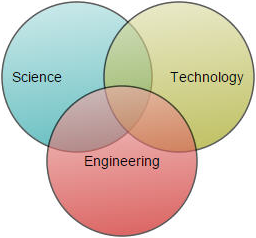## Create Node

A node can be created and added to the Diagram, either programmatically or interactively. Nodes are stacked on the Diagram area from bottom to top in the order they are added.

### Add node through nodes collection

To create a node, You have to define the node object and add that to `nodes` collection of the Diagram model. The following code example illustrates how to add a node to the Diagram.

• HTML
• ``````<div ng-controller="diagramCtrl">
<ej-diagram id="diagram" e-height="600px" e-width="100%" e-nodes="nodes">
</ej-diagram>
</div>``````
• JAVASCRIPT
• ``````var nodes = [{
name: "node1",
width: 100,
height: 100,
offsetX: 250,
offsetY: 250,
fillColor: "darkcyan",
borderWidth: 2
}, ];

syncApp.controller('diagramCtrl', function(\$scope) {
\$scope.nodes = nodes;
});``````## Position

Position of a node is controlled by using its `offsetX` and `offsetY` properties. By default, these offset properties represent the distance between origin of the Diagram’s page and node’s center point. You may expect this offset values to represent the distance between page origin and node’s top left corner instead of center. `pivot` property helps solve this problem. Default value of node’s pivot point is (0.5, 0.5), that means center of Node.

The following table illustrates how pivot relates offset values with node boundaries.

Pivot Offset
(0.5,0.5) offsetX and offsetY values are considered as the node’s center point.
(0,0) offsetX and offsetY values are considered as the top left corner of node
(1,1) offsetX and offsetY values are considered as the bottom right corner of the node.

The following code illustrates how to change the `pivot` value.

• HTML
• ``````<div ng-controller="diagramCtrl">
<ej-diagram id="diagram" e-height="600px" e-width="100%" e-nodes="nodes">
</ej-diagram>
</div>``````
• JAVASCRIPT
• ``````var nodes = [{
name: "node1",
width: 100,
height: 100,
offsetX: 250,
offsetY: 250,
shape: "rectangle",
pivot: ej.datavisualization.Diagram.Point(0, 0)
}, ];
syncApp.controller('diagramCtrl', function(\$scope) {
\$scope.nodes = nodes;
});``````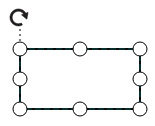## Types

Diagram allows to add different kind of nodes. To explore the types of nodes, refer to Types of Nodes.

## Appearance

You can customize the appearance of a node by changing its font, fill colors, patterns, line weight and style, or shadow. The following code illustrates how to customize the appearance of the shape.

• HTML
• ``````<div ng-controller="diagramCtrl">
<ej-diagram id="diagram" e-height="600px" e-width="100%" e-nodes="nodes">
</ej-diagram>
</div>``````
• JAVASCRIPT
• ``````var nodes = [{
name: "node1",
width: 100,
height: 100,
offsetX: 250,
offsetY: 250,
fillColor: "darkcyan",
borderWidth: 2,
borderColor: "black",
borderDashArray: "5 5",
}, ];
syncApp.controller('diagramCtrl', function(\$scope) {
\$scope.nodes = nodes;
});``````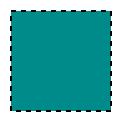There are two types of gradients.

• Linear gradient - Defines a smooth transition between a set of colors (so-called “stops”) on a line.
• Radial gradient - Defines a smooth transition between stops on a circle.

The `gradient` property of node allows you to define and applies the gradient effect to that node.

• HTML
• ``````<div ng-controller="diagramCtrl">
<ej-diagram id="diagram" e-height="600px" e-width="100%" e-nodes="nodes">
</ej-diagram>
</div>``````
• JAVASCRIPT
• ``````//Creates linear gradient
type: "linear",
x1: 0,
y1: 0,
x2: 50,
y2: 50,
//Sets an array of stop objects
stops: [{
color: "white",
offset: 0
},
{
color: "darkCyan",
offset: 100
}
]
};

//Center point of outer circle
cx: 50,
cy: 50,
//Center point of inner circle
fx: 25,
fy: 25,
r: 50,
//Sets an array of stop objects
stops: [{
color: "white",
offset: 0
},
{
color: "darkCyan",
offset: 100
}
]
};

var nodes = [{
name: "node1",
width: 100,
height: 100,
offsetX: 250,
offsetY: 250,
//Sets styles to a node to customize the appearance
fillColor: "darkcyan",
borderWidth: 2,
}];
syncApp.controller('diagramCtrl', function(\$scope) {
\$scope.nodes = nodes;
});``````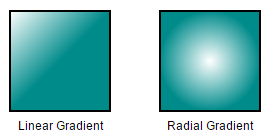Diagram provides support to add shadow effect to a node that is disabled by default. It can be enabled with the `constraints` property of node. The following code illustrates how to drop shadow.

• JAVASCRIPT
• ``````var nodeConstraints = ej.datavisualization.Diagram.NodeConstraints;

//Enables Shadow effect for a node.
var constraints = nodeConstraints.Default | nodeConstraints.Shadow;

// Defines JSON to create path node
var nodes = [{
name: "node",
offsetX: 100,
offsetY: 100,
height: 100,
width: 100,
//Sets shape of node
shape: "rectangle",
constraints: constraints
}];
syncApp.controller('diagramCtrl', function(\$scope) {
\$scope.nodes = nodes;
});``````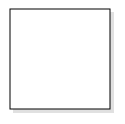The following code illustrates how to disable shadow effect at runtime.

• JAVASCRIPT
• ``````var nodeConstraints = ej.datavisualization.Diagram.NodeConstraints;

//Enables Shadow effect for a node.
var constraints = nodeConstraints.Default & ~nodeConstraints.Shadow;

// Defines JSON to create path node
var nodes = [{
name: "node",
offsetX: 100,
offsetY: 100,
height: 100,
width: 100,
//Sets shape of node
shape: "rectangle",
constraints: constraints
}];
syncApp.controller('diagramCtrl', function(\$scope) {
\$scope.nodes = nodes;
});``````

The angle, translation, and opacity of the shadow can be customized with the `shadow` property of node. The following code example illustrates how to customize shadow.

• JAVASCRIPT
• ``````var nodes = [{
name: "node",
offsetX: 100,
offsetY: 100,
height: 100,
width: 100,
//Sets shape of node
shape: "rectangle",
constraints: constraints,
opacity: 0.8,
distance: 9,
angle: 50
}
}];
syncApp.controller('diagramCtrl', function(\$scope) {
\$scope.nodes = nodes;
});``````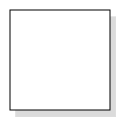## Icon

Diagram provides support to describe the state of the node. i.e., node is expanded or collapsed state.

NOTE

Icon can be created only when the node has outedges.

To explore the properties of expandIcon and collapseIcon, refer to Icon Properties.

The `shape` property of icon allows to define the shape of the icon. The following code example illustrates how to create icon of various shapes.

• HTML
• ``````<div ng-controller="diagramCtrl">
<ej-diagram id="diagram" e-height="600px" e-width="100%" e-nodes="nodes" e-connectors="connectors">
</ej-diagram>
</div>``````
• JAVASCRIPT
• ``````var nodes = [{
name: "node1",
width: 100,
height: 100,
offsetX: 100,
offsetY: 100,
shape: "rectangle",
expandIcon: {
shape: "arrowdown",
width: 10,
height: 10,
},
collapseIcon: {
shape: "arrowup",
width: 10,
height: 10,
}
},
{
name: "node2",
offsetX: 100,
offsetY: 100,
height: 100,
width: 100,
shape: "rectangle"
}
];
var connectors = [{
name: "connect",
sourceNode: "node1",
targetNode: "node2"
}];
syncApp.controller('diagramCtrl', function(\$scope) {
\$scope.nodes = nodes;
\$scope.connectors = connectors;

});``````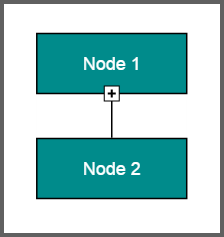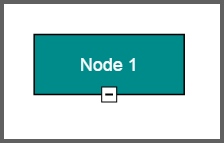## Interaction

Diagram provides support to drag, resize, or rotate the node interactively. For more information about editing a node at runtime, refer to Edit Nodes.

## Constraints

The `constraints` property of node allows you to enable/disable certain features. For more information about node constraints, refer to Node Constraints.